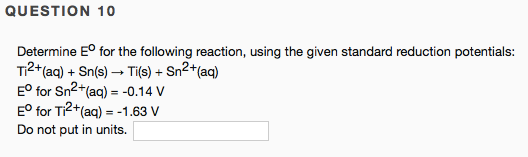# Determine E° for the following reaction, using the given standard reduction potentials: Ti^2+(aq) + Sn(s) → Ti(s) + Sn^2+(aq) E° for Sn^2+(aq) = -0.14 V; E° for Ti^2+(aq) = -1.63 V. Do not put in units.